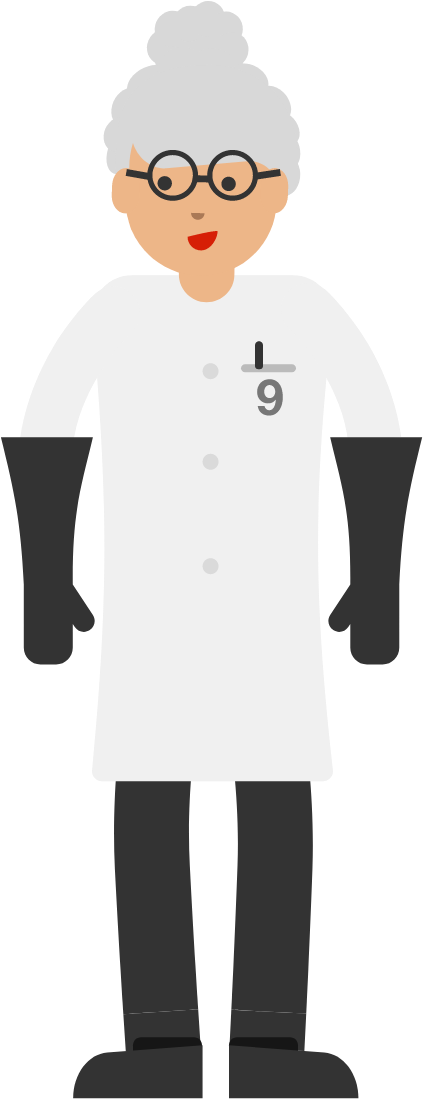Reversal NinesDr. Frankenine (cousin of Dr. Frankenthree) took positive integers, reversed their digits, and subtracted the two numbers. She noticed all the results were divisible by 9: \begin{aligned} {\color{#3D99F6}83}-{\color{#20A900}38} &= 45 \\&= 9 \times 5 \\\\ {\color{#3D99F6}113}-{\color{#20A900}311} &= -198 \\&= 9 \times (-22) \\\\ {\color{#3D99F6}1431} -{\color{#20A900}1341} &= 90 \\&= 9 \times 10 \\\\ {\color{#3D99F6}44344} -{\color{#20A900}44344} &= 0 \\&= 9 \times 0. \end{aligned} Are the numbers Dr. Frankenine creates in this way always multiples of 9?

Note: This puzzle is in base 10.

×